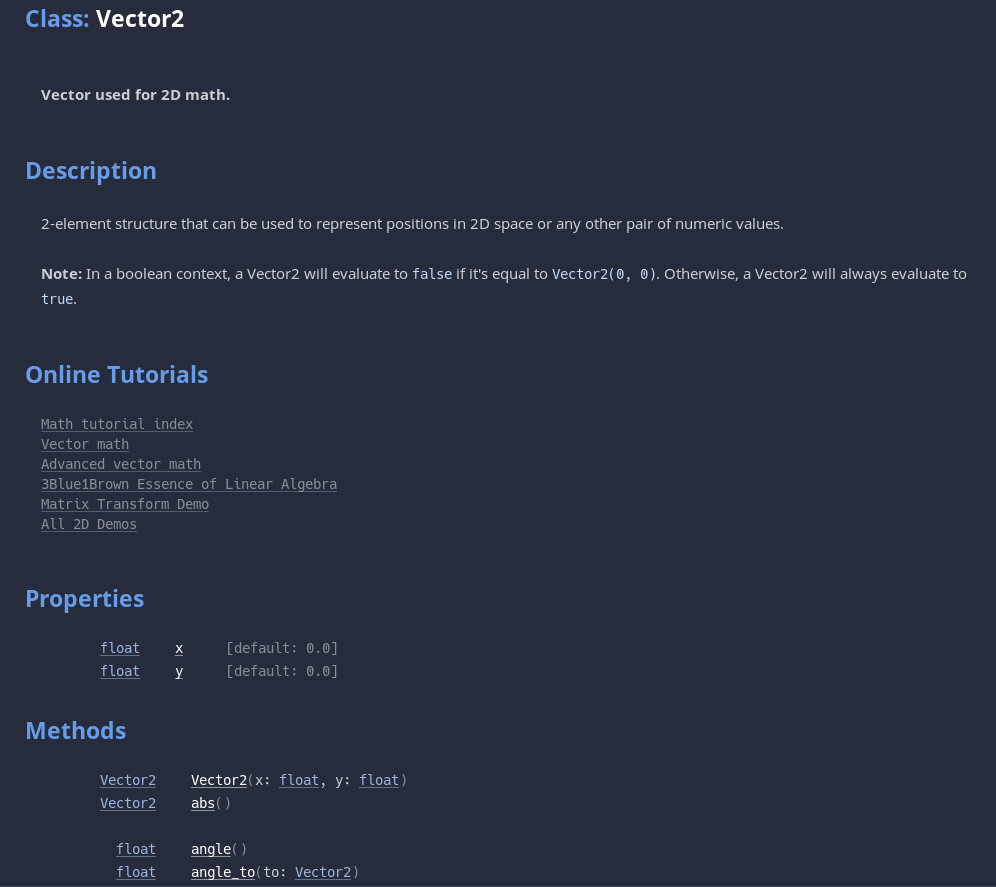r
rifqilubis

# Predefined variable

func _process(delta: float) -> void:

var direction := Vector2.ZERO

direction.x = Input.get_axis("move_left", "move_right")

direction.y = Input.get_axis("move_up", "move_down")

the direction.x and direction.y   are not predefined variable right?

i mean, i see other tutorials uses input_x and input_y for the naming.

if direction.x and y are not predefined variable.

then how godot know that direction.x is for horizontal movement?

•Solution

Actually they are predefined. So whenever we use a framework or library or game engine in this case, the purpose of using these is that they bring a lot of functionality out of the box that we don't need to construct ourselves, such as functions, algorithms, objects etc.

In this case we use the Vector2 Godot built-in object that have a number of properties. These properties are variables defined for us by the engine, which include x & y among others. We could have used individual variables as you mention, like input_x & input_y etc.

The benefit of using built-in objects is they give us extra properties and functionalities that we might need in our projects, such as Vector2 scalar multiplication, Vector2 & Vector2 addition, multiplication and other operations, direction.length(), direction.normalized() etc. that we don't have to code ourselves.

You can always bring up the help system with F1 to see the list of defined variables (properties) and functions (methods) that the object pre-defines for us:• r
rifqilubis replied

i saw other people using input and vec as their variable name. so when people define variable for example:

var vec := Vector2.ZERO

that means you can use vec as the main variable for x and y properties right?

vec.x = Input.get_axis("move_left", "move_right")

vec.y = Input.get_axis("move_up", "move_down")

this is correct right?

so the "direction" variable are not predefined variable(?)

• r
rifqilubis replied

because i can't find direction as predefined variable in the godot documentation

•Solution

@rifqilubis yes, thst's correct. Any statement that starts with var variable_name defines variable_name which can be anything that follows the allowed nomenclature (e.g. it can't start with a digit for example etc.). So all these:

```var vec := Vector2.ZERO
var some_other_vec := Vector2.ONE
var some_flag := false```

All of these, vec, some_other_vec, some_flag are defined variables by us and direction is one of them.

The defined names are just for us programmers to keep track of our coding and possibly for team mates or any other programmer.

The computer doesn't care about it, we could as well just say var d := Vector2.ZERO etc. but that wouldn't be very useful for teaching purposes and even for ourselves to keep track of our own code.

1 love
• r
rifqilubis replied

thanks